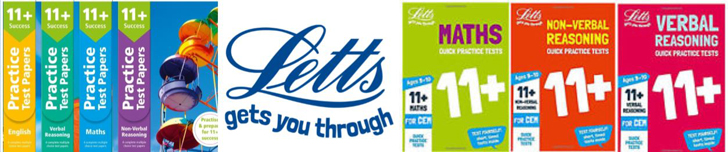# Multiplication Tips and Tricks

## Even Numbers

If a number is even, ending in 0, 2, 4, 6 or 8, and it is multiplied by any number,
the result will always be even.

## Odd Numbers

If a number is odd, ending in 1, 3, 5, 7 or 9, and it is multiplied by another odd number,
the result will always be odd.

### 3 x 1 =3 ... 7 x 3 = 21 ... 313 x 7 = 2191

If a number is odd, ending in 1, 3, 5, 7 or 9, and it is multiplied by an even number,
the result will always be even.

## Multiplying by 0

Multiply any number by zero and the result is always zero.
If a person gave you £0 every day for a year - you would still have £0.

## Multiplying by 1

Any number multiplied by 1 is always itself.

## Multiplying by 2

Is the same as doubling - just add it to itself.

## Multiplying by 4

Is the same as multiplying by 2 and then multiplying by 2 again.

Double the number and then double the answer.

## Multiplying by 5

If the number is even, then halve it and times by 10.

### 4 x 5 = (4 ÷ 2) x 10 = 2 x 10 = 20

This also works for odd numbers, but you will need to use decimals.

### 7 x 5 = (7 ÷ 2) x 10 = 3.5 x 10 =35

or for odd numbers, subtract 1 from the number, halve it and then put a 5 next to it.

## Multiplying by 6

Multiplying by 6 is the same as multiplying by 3 and doubling the answer.

## Multiplying by 7

There are no tricks that I know of for multiplying by 7 - email me if you know of one!

This rhyme may help for 7 x 7 ... "Seven times seven is forty nine and your doing fine"

for 8 x 7, remember the sequence 5,6,7,8 ... 56 = 7 x 8

## Multiplying by 8

Multiplying by 8 is the same as multiplying by 4 and doubling the answer.

### 5 x 8 = (5 x 4) x 2 = 20 x 2 = 40

One rhyme that all kids remember is "I ate and I ate t'ill I was sick on the floor"
"Eight eights are sixty four"

## Multiplying by 9

Most children think that this is a difficult one to remember, so try this.

Put both hands in front of you, palms up. Number your fingers and thumbs from left to right.

To multiply 3 x 9 - curl up your finger 3 (which is the middle finger of your left hand).
You have 2 fingers to the left of it and 7 to the right - 2 and 7 ... 27.

To multiply 6 x 9 - curl up your finger 6 (which is the little finger of your right hand).
You have 5 fingers to the left of it and 4to the right - 4 and 5 ... 45.

or

Take the number that you want to multiply 9 by and subtract 1, this gives you the first digit of the answer - then work out what you need to add to this figure to make it 9 and this is the second digit of the answer.

9 x 7:    7 - 1 = 6 (1st digit of answer) ... you need to add 3 to 6 to make 9 (3 is the 2nd digit)

or

Calculate 10 times the number and then take away the number.

### 9 x 8:   10 x 8 = 80, 80 -8 = 72

To check, add the answers digits together to form a single number, that number will be 9.

## Multiplying by 10

The simple way is just to place a zero after the number

To multiply by 10, move all the digits 1 place to the left (see place value)

## Multiplying by itself

A number that is multiplied by the same number is called a square number.

It will be worthwhile remembering the square numbers.